# Python时间序列分析--从线性模型到GARCH模型

2017/06/28 22:41

1.平稳性
2.序列相关（自相关）
3.为什么我们关心序列相关性？

## 一、Motivation

``````                       **时间序列分析试图明白过去并且预测未来**
``````

Before we begin let’s import our Python libraries.

``````import os
import sys

import pandas as pd
import numpy as np

import statsmodels.formula.api as smf
import statsmodels.tsa.api as smt
import statsmodels.api as sm
import scipy.stats as scs
from arch import arch_model

import matplotlib.pyplot as plt
import matplotlib as mpl
%matplotlib inline
p = print

p('Machine:{} {}\n'.format(os.uname().sysname,os.uname().machine))
p(sys.version)``````
•

Let’s use the pandas_datareader package to grab some sample data using the Yahoo Finance API.

``````start = '2010-01-01'
end = '2017-02-25'
get_px = lambda x: web.get_data_yahoo(x, start=start, end=end)['Adj Close']

symbols = ['SPY', 'TLT', 'MSFT']

data = pd.DataFrame({sym:get_px(sym) for sym in symbols})

Irets = np.log(data/data.shift(1)).dropna()``````
•

## 二、基础知识

What is a Time Series? A time series is a series of data points indexed (or listed or graphed) in time order. - Wikipedia
1.平稳性

a.序列的平均值不应该是时间的函数。下图中红色的线是不平稳的，因为它的平均值随时间增长。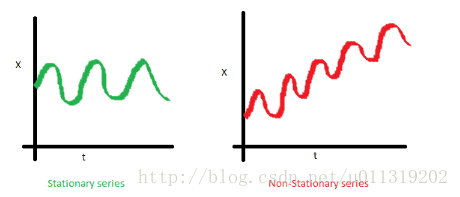b.序列的方差不应该是时间的函数。这个属性被称为同方差。注意到红色数据随时间的变化很大。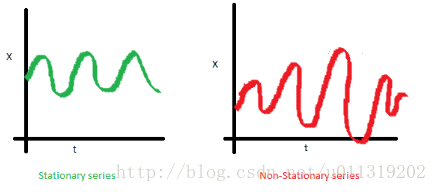c.最后，第i项和第i+m项的协方差不应该是时间的函数。在下面的图中，你会注意到随时间的推移差距变得越来越近。因此，红色序列的协方差是随时间变化的。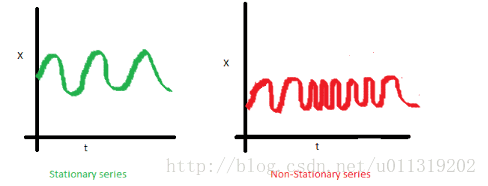So what? Why do we care about stationarity?

• 一个平稳的时间序列是容易进行预测的，我们可以假设其未来与现在的统计属性是相同的或成比例的。
• 我们用的大多数时间序列分析模型都是假设协方差平稳。这就意味着这些预测模型的统计描述如均值、方差、协方差等只有在序列平稳时才是有效的，否则无效。

2.序列相关（自相关）–Serial Correlation (Autocorrelation)

3.为什么我们关心序列相关性？–Why Do We Care about Serial Correlation?

## 三、白噪声和随机游动–White Noise and Random Walks

``````def tsplot(y, lags=None, figsize=(10,8), style='bmh'):
if not isinstance(y, pd.Series):
y = pd.Series(y)
with plt.style.context(style):#定义局部样式
fig = plt.figure(figsize=figsize)
layout = (3, 2)
ts_ax = plt.subplot2grid(layout, (0, 0), colspan=2)
acf_ax = plt.subplot2grid(layout, (1,0))
pacf_ax = plt.subplot2grid(layout, (1,1))
qq_ax = plt.subplot2grid(layout, (2,0))
pp_ax = plt.subplot2grid(layout, (2,1))

y.plot(ax=ts_ax)
ts_ax.set_title('Time Series Analysis Plots')
smt.graphics.plot_acf(y, lags=lags, ax=acf_ax, alpha=0.5)#自相关系数ACF图
smt.graphics.plot_pacf(y, lags=lags, ax=pacf_ax, alpha=0.5)#偏相关系数PACF图
sm.qqplot(y, line='s', ax=qq_ax)#QQ图检验是否是正太分布
qq_ax.set_title('QQ Plot')
scs.probplot(y, sparams=(y.mean(), y.std()), plot=pp_ax)

plt.tight_layout()
return``````
• We can model a white noise process easily and output the TS plot for visual inspection.
``````np.random.seed(1)
randser = np.random.normal(size=1000)#高斯概率分布
tsplot(randser, lags=30)``````
•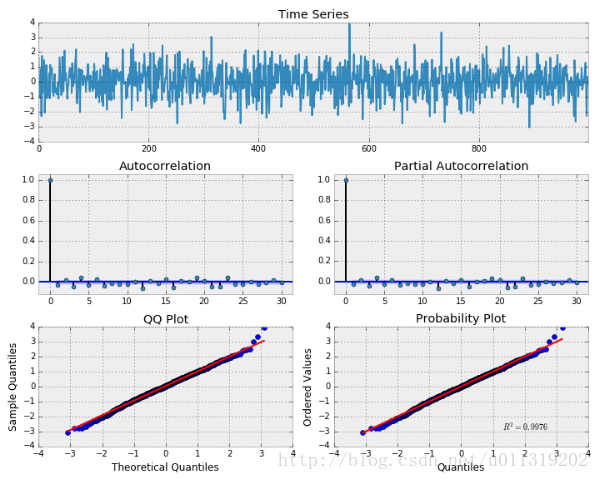``````p("Random Series\n------------\nmean: {:.3f}\nvariance: {:.3f}\nstandard deviation: {:.3f}"
.format(randser.mean(), randser.var(), randser.std()))
# Random Series
# -------------
# mean: 0.039
# variance: 0.962
# standard deviation: 0.981``````
•

A Random Walk is defined below: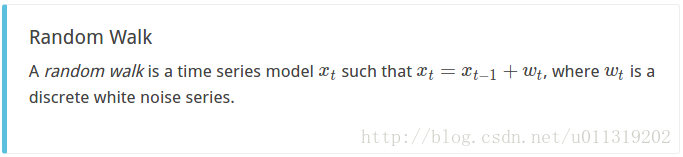``````#Random Walk without a drift
np.random.seed(1)
n_samples = 1000

x = w =np.random.normal(size=n_samples)
for t in range(n_samples):
x[t] = x[t-1] + w[t]
_ = tsplot(x, lags=30)``````
•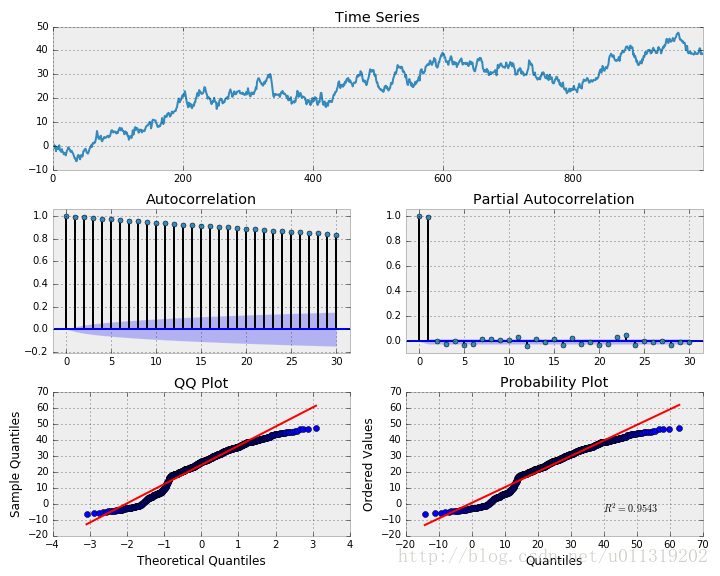``````#First difference of simulated Random Walk series
#随机游走序列的一阶差分
_ = tsplot(np.diff(x),lags=30)``````
•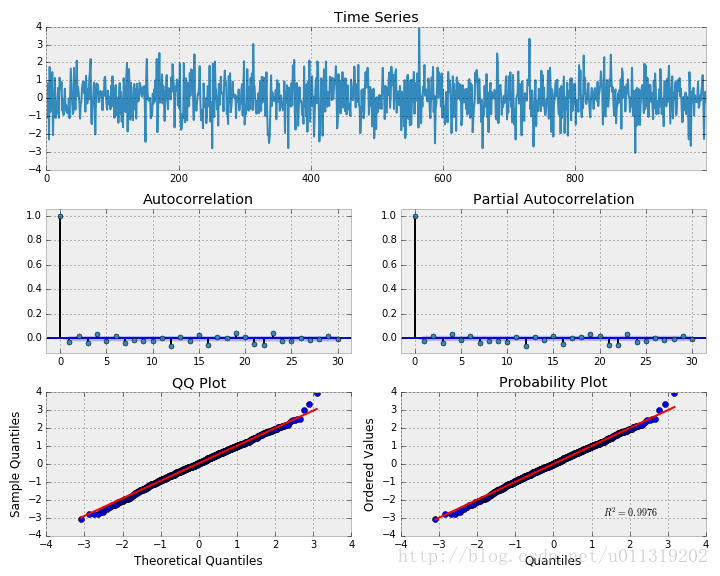Our definition holds as this looks exactly like a white noise process. What if we fit a random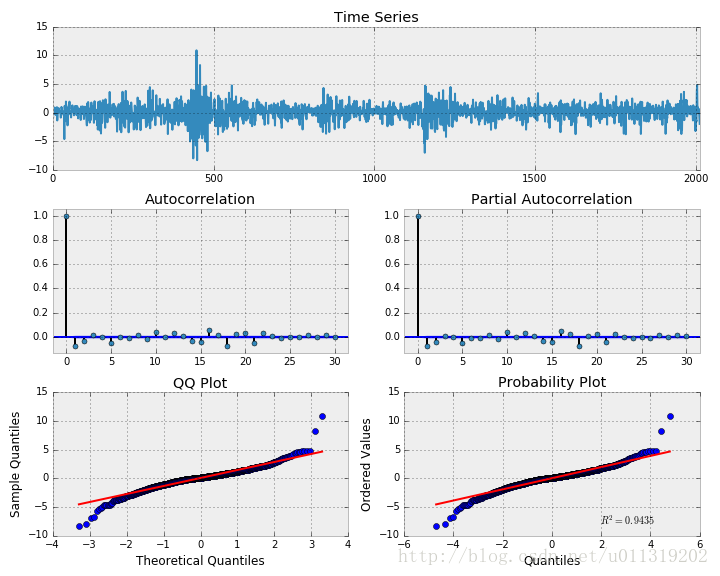0
0 收藏

### 作者的其它热门文章0 评论
0 收藏
0